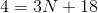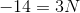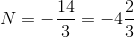## Example Questions

### Example Question #1 : How To Graph A Line

What is the distance between (7, 13) and (1, 5)?

12

None of the answers are correct

7

5

10

10

Explanation:

The distance formula is given by d = square root [(x2 – x1)2 + (y2 – y1)2].  Let point 2 be (7,13) and point 1 be (1,5).  Substitute the values and solve.

### Example Question #2 : How To Graph A Line

What is the slope of this line?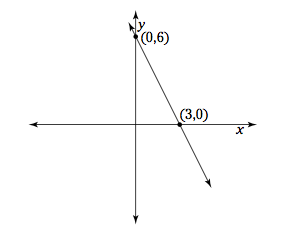Explanation:

The slope is found using the formula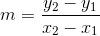.

We know that the line contains the points (3,0) and (0,6). Using these points in the above equation allows us to calculate the slope.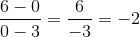### Example Question #3 : How To Graph A Line

What is the amplitude of the function if the marks on the y-axis are 1 and -1, respectively?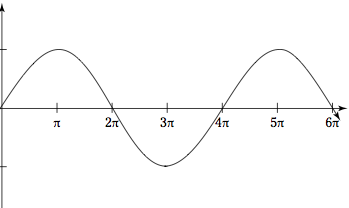1

2π

π

0.5

3π

1

Explanation:

The amplitude is half the measure from a trough to a peak.

### Example Question #4 : How To Graph A Line

What is the midpoint between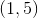and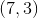?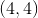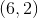None of the answers are correct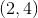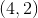Explanation:

The x-coordinate for the midpoint is given by taking the arithmetic average (mean) of the x-coordinates of the two end points. So the x-coordinate of the midpoint is given by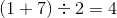The same procedure is used for the y-coordinates. So the y-coordinate of the midpoint is given by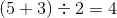Thus the midpoint is given by the ordered pair### Example Question #5 : How To Graph A Line

If the graph has an equation of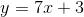, what is the value of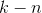?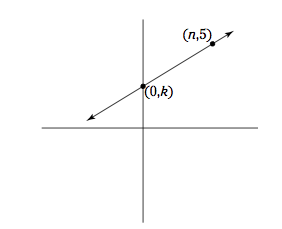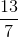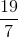Explanation:is the-intercept and equalscan be solved for by substitutingin the equation for, which yields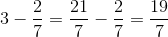### Example Question #671 : Sat Mathematics

The equation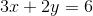represents a line.  This line does NOT pass through which of the four quadrants?

III

I

Cannot be determined

IV

II

III

Explanation:

Plug inforto find a point on the line: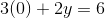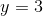Thus,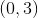is a point on the line.

Plug inforto find a second point on the line: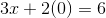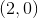is another point on the line.

Now we know that the line passes through the pointsand.

A quick sketch of the two points reveals that the line passes through all but the third quadrant.

### Example Question #1 : Graphing Linear Functions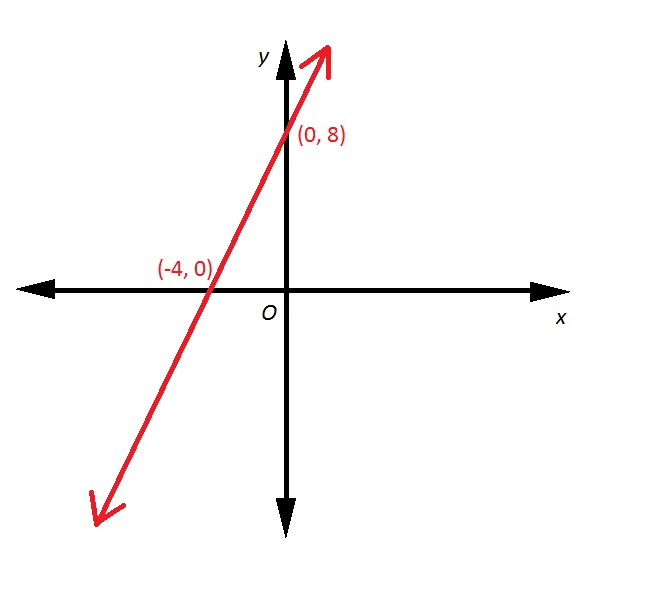Refer to the above red line. A line is drawn perpendicular to that line, and with the same-intercept.  Give the equation of that line in slope-intercept form.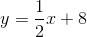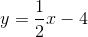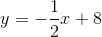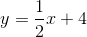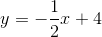Explanation:

First, we need to find the slope of the above line.

The slope of a line. given two points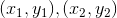can be calculated using the slope formulaSet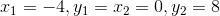: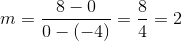The slope of a line perpendicular to it has as its slope the opposite of the reciprocal of 2, which would be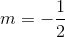. Since we want this line to have the same-intercept as the first line, which is the point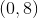, we can substituteand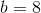in the slope-intercept form:### Example Question #2 : Graphing Linear Functions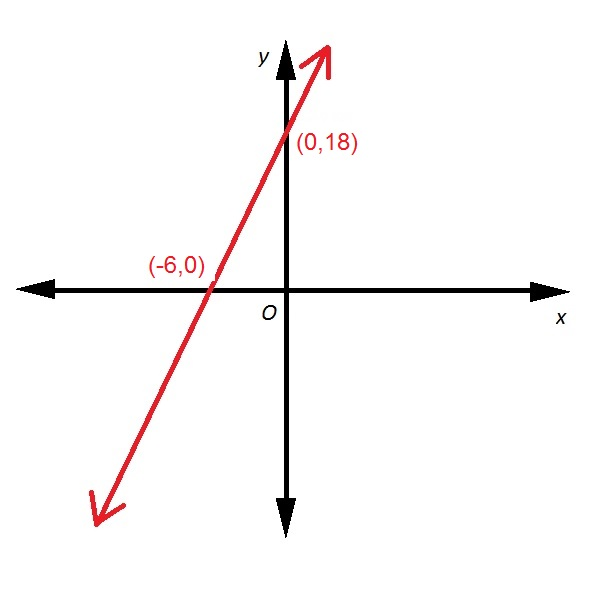Refer to the above diagram. If the red line passes through the point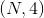, what is the value of?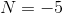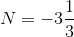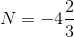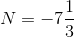Explanation:

One way to answer this is to first find the equation of the line.

The slope of a line. given two pointscan be calculated using the slope formulaSet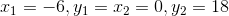: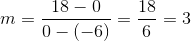The line has slope 3 and-intercept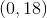, so we can substitute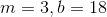in the slope-intercept form: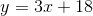Now substitute 4 forandforand solve for: